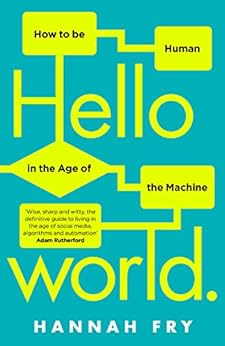Can you use the digits on the left of this clock along with any mathematical operations to equal the digits on the right (also with any mathematical operations)?

## ExamplesWhich times is this possible and which times impossible?

## Alternate activity

Use the digits of the current time to make as many different calculations as possible all with different answers.

All four digits must be used and each must appear once only in each of your calculations.

Next choose a different time that you think will produce more calculations than the present time.

## A Mathematics Lesson Starter Of The Day

Share
• Transum,
•
• The key to making this activity a successful starter is not just considering the current time but focussing on the question 'Which times is this possible and which times impossible?'. The question 'How many times can you find that are possible?' might lead to a list of similar mathematical operations (which is fine for some pupils) while dividing the class into small groups and assigning each group an hour of the day may encourage some more creativity. Let us know if your class make any interesting discoveries.

How did you use this starter? Can you suggest how teachers could present or develop this resource? Do you have any comments? It is always useful to receive feedback and helps make this free resource even more useful for Maths teachers anywhere in the world.

If you don't have the time to provide feedback we'd really appreciate it if you could give this page a score! We are constantly improving and adding to these starters so it would be really helpful to know which ones are most useful. Simply click on a button below:

Excellent, I would like to see more like this
Good, achieved the results I required
Satisfactory
Didn't really capture the interest of the students
Not for me! I wouldn't use this type of activity.

This starter has scored a mean of 3.0 out of 5 based on 277 votes.

Previous Day | This starter is for 14 June | Next Day

 09 01 0x9=0 0x1=0 09 02 0x9=0 0x2=0 09 03 0x9=0 0x3=0 09 04 0x9=0 0x4=0 09 05 0x9=0 0x5=0 09 06 0x9=0 0x6=0 09 07 0x9=0 0x7=0 09 08 0x9=0 0x8=0 09 09 90 90 09 10 0x9=0 1x0=0 09 11 90=1 11=1 09 12 90=1 12=1 09 13 90=1 13=1 09 14 90=1 14=1 09 15 90=1 15=1 09 16 90=1 16=1 09 17 90=1 17=1 09 18 90=1 18=1 09 19 90=1 19=1 09 20 0x9=0 0x2=0 09 21 √9+0=3 2+1=3 09 22 90=1 2÷2=1 09 23 0+9=9 32=9 09 24 √9+0=3, 3!=6 2+4=6 09 25 √9+0=3 5-2=3 09 26 √9+0=3 6÷2=3 09 27 0+9=9 2+7=9 09 28 90=1 82=64, 6+4=10, 1+0=1 09 29 0+9=9 92=81, 8+1=9 09 30 0x9=0 0x3=0

For more ideas see Alan Sturgess' Maths Puzzle Investigation video.

Your access to the majority of the Transum resources continues to be free but you can help support the continued growth of the website by doing your Amazon shopping using the links on this page. Below is an Amazon search box and some items chosen and recommended by Transum Mathematics to get you started.

## Hello World

You are buying a (driverless) car. One vehicle is programmed to save as many lives as possible in a collision. Another promises to prioritize the lives of its passengers. Which do you choose?

Welcome to the age of the algorithm, the story of a not-too-distant future where machines rule supreme, making important decisions – in healthcare, transport, finance, security, what we watch, where we go even who we send to prison. So how much should we rely on them? What kind of future do we want?

Hannah Fry takes us on a tour of the good, the bad and the downright ugly of the algorithms that surround us. In Hello World she lifts the lid on their inner workings, demonstrates their power, exposes their limitations, and examines whether they really are an improvement on the humans they are replacing. more...Teacher, do your students have access to computers?Do they have iPads or Laptops in Lessons? Whether your students each have a TabletPC, a Surface or a Mac, this activity lends itself to eLearning (Engaged Learning).Here a concise URL for a version of this page without the comments.

Transum.org/go/?Start=June14

Here is the URL which will take them to a related student activity.

Transum.org/go/?to=fifteen